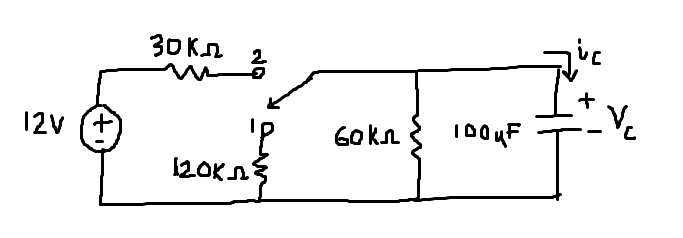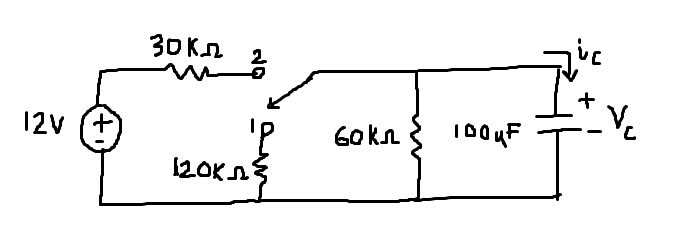# Response of RC circuit i/v of capacitor help

• Engineering

## Homework Statementafter having been in position 1 for a long time, the switch was moved to position 2 at t=0. determine:
a) i-c(0-) and vc(0-)
b) i-c(0) and v-c(0)
c) i-c(infinity) and v-c(infinity)
d) v-c(t) for t>=0
e) i-c(t) for t >=0

## The Attempt at a Solution

not sure how to find voltages/current of capacitor. i have general equations i think though:

for t=0- (before t=0): v(t)= v(0)e^-t/(RC) = 12e^(-t/4)
i(t)= -Vs/R*(e^-t/(RC))= 300uI*e^-t/4

for t=0 (after t=0): not sure exactly how to find v(t) (which i think in this case is also v(infinity)), but i made an attempt:
find voltage across 60k ohm resistor by doing voltage division, 12(60k/(60k+30k))=8V
v(t)=8e^-t/(RC)
not sure how to find the eq. resistance in this one. should i do 30 || 60?

Last edited:

berkeman
Mentor

## Homework Statementafter having been in position 1 for a long time, the switch was moved to position 2 at t=0. determine:
a) i-c(0-) and vc(0-)
b) i-c(0) and v-c(0)
c) i-c(infinity) and v-c(infinity)
d) v-c(t) for t>=0
e) i-c(t) for t >=0

## The Attempt at a Solution

not sure how to find voltages/current of capacitor. i have general equations i think though:

for t=0- (before t=0): v(t)= v(0)e^-t/(RC) = 12e^(-t/4)
i(t)= -Vs/R*(e^-t/(RC))= 300uI*e^-t/4

for t=0 (after t=0): not sure how to find v(t) because the 60k(ohm) is not in series with the capacitor.

For t=0-, what is the voltage on the capacitor? The voltage source has been disconnected for a long time....

And for t>0, you should write a KCL equation at the top/middle node, and use the equation that relates the current through the capacitor to the change in voltage across it I = C dv/dt.

Solve the differential equation (you get exponential functions like you indicate), and use the initial condition for the voltage on the cap at t=0-.

For t=0-, what is the voltage on the capacitor? The voltage source has been disconnected for a long time....

And for t>0, you should write a KCL equation at the top/middle node, and use the equation that relates the current through the capacitor to the change in voltage across it I = C dv/dt.

Solve the differential equation (you get exponential functions like you indicate), and use the initial condition for the voltage on the cap at t=0-.

well then v-c(0-) must be 0V then right? but according to my teacher voltage never changes instantaneously, so v(0-)=v(0)=V(s)=12V so how can this be?

and I do the KCL for the top node for t>=0 like you said but it's turning out to be a mess, think i did it wrong:

(V-12)/30k + V/120k+ V/60k - i(c) = 0
(7/120)V-0.4=C dv/dt
((7/120)V/C)-(0.4/C)=dv/dt
dv/dt - a*(7/120)V + 0.4a = 0 where a=1/C
dv/dt(e^at) - (7/120)av(e^at) + 0.4a(e^at)=0

berkeman
Mentor
well then v-c(0-) must be 0V then right? but according to my teacher voltage never changes instantaneously, so v(0-)=v(0)=V(s)=12V so how can this be?

and I do the KCL for the top node for t>=0 like you said but it's turning out to be a mess, think i did it wrong:

(V-12)/30k + V/120k+ V/60k - i(c) = 0
(7/120)V-0.4=C dv/dt
((7/120)V/C)-(0.4/C)=dv/dt
dv/dt - a*(7/120)V + 0.4a = 0 where a=1/C
dv/dt(e^at) - (7/120)av(e^at) + 0.4a(e^at)=0

Correct that Vc(t=0-)=0V. And also correct that the voltage across a capacitor cannot change instantaneously.

After the switch changes at t=0, the 120k resistor is out of the picture, so delete it from your KCL.

Once you have your Differential Equation (without the 120k), what solution are you assuming for the V(t)? And what is the derivative of that V(t)? If you could make it a bit more clear how you are solving the DE and imposing the initial conditions, that would help.

yes youre right, my mistake. (V-12)/30k+V/60k=i(C)
(1/20)V-0.4=C(dv/dt)
dividing both sides by C: (1/20)V/C - 0.4/C = dv/dt
dv/dt - (1/20)av + 0.4a = 0 where a=1/C
multiply both sides by e^at: (dv/dt)e^at - (0.05av)e^at + (0.4a)e^at=0

i think this is right, but i have no idea how to simplify or differentiate this.